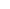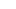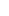# Forex Smart Trade Results, Friday, September 1, 2023 – \$10,853###### Forex Smart Trade Results, Thursday, August 31, 2023 – \$11,151
September 4, 2023###### Forex Smart Trade Results, Monday, September 4, 2023 – \$2,229
September 9, 2023

# Know the 3 Other Types of Pivot Points.

Know the 3 other types of pivot points.
The standard method of calculating pivot points is NOT the only way to calculate pivot points.

Just like listening to BTS is not the only way to listen to K-pop.

Traders have worked on improving the original pivot point and now there are other ways to calculate for pivot points.

In this lesson, we will talk about these other methods, as well as give you the formulas on how to calculate these levels.

## Woodie Pivot Point

R2 = PP + High – Low

R1 = (2 X PP) – Low

PP = (H + L + 2C) / 4

S1 = (2 X PP) – High

S2 = PP – High + Low

C – Closing Price, H – High, L – Low

In the formulas above, you’ll notice that the pivot point calculation is very different from the standard method.

Also, in order the calculate the corresponding support and resistance levels, you would use the difference between the previous day’s high and low, otherwise known as the range.

## Example

Here’s a chart example of the Woodie pivot point calculation applied on EURUSD.

The Woodie pivot point, support levels, and resistance levels are the solid lines while the dotted lines represent the levels calculated through the standard method.Because they have different formulas, levels obtained through the Woodie calculations are very different from those obtained through the standard method.

Some traders prefer to use the Woodie formulas because they give more weight to the closing price of the previous period.Others prefer the standard formulas because many traders make use of those which could make them self-fulfilling.

In any case, since resistance turns into support (and vice versa), if you choose to use the Woodie formulas, you should keep an eye on these levels, as they could become areas of interest.